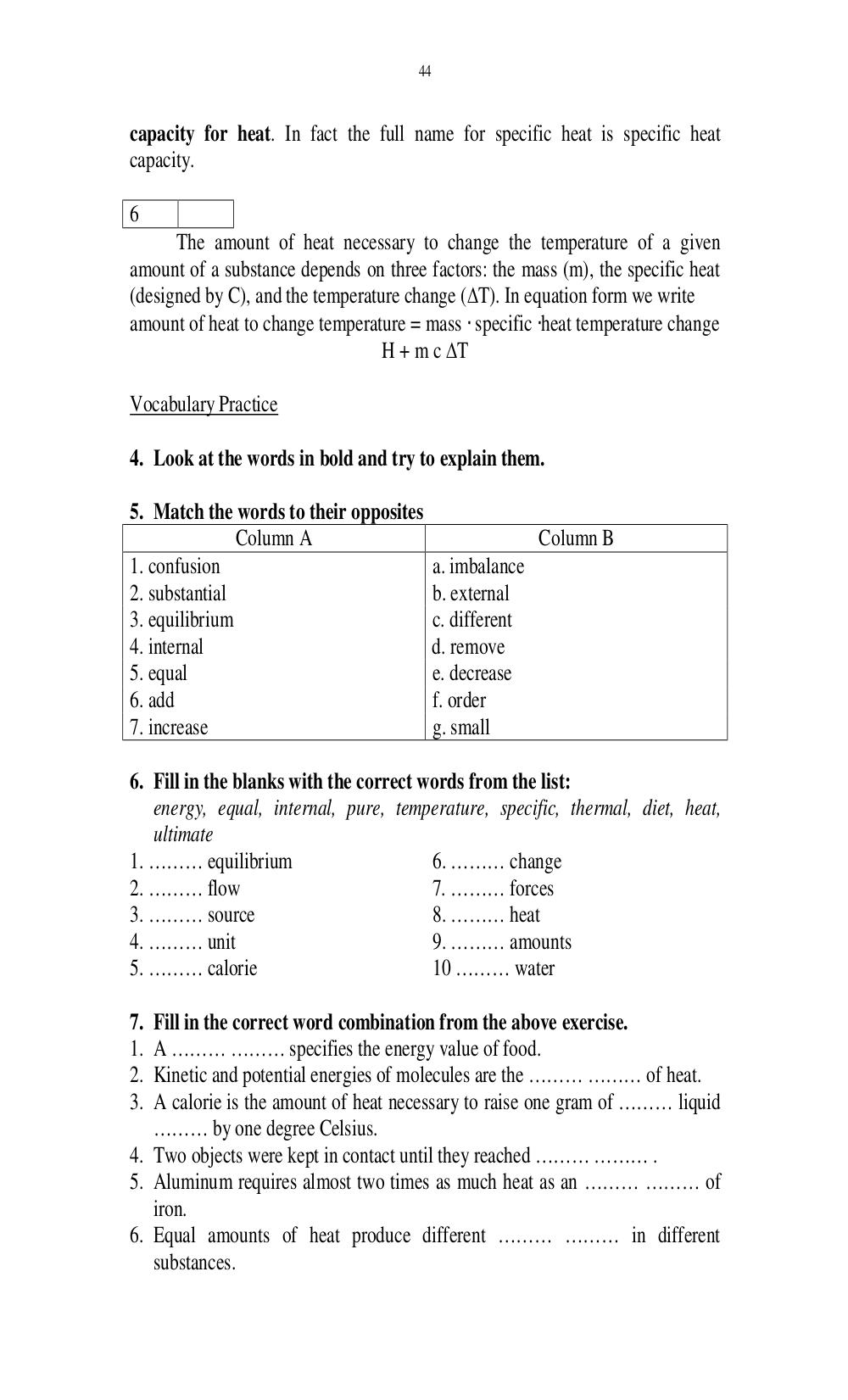# Английский язык. Ильичева Н.А - 44 стр.

Составители:

Рубрика:

• ## Иностранный язык44
capacity for heat. In fact the full name for specific heat is specific heat
capacity.
6
The amount of heat necessary to change the temperature of a given
amount of a substance depends on three factors: the mass (m), the specific heat
(designed by C), and the temperature change (T). In equation form we write
amount of heat to change temperature = mass specific heat temperature change
H + m c T
Vocabulary Practice
4. Look at the words in bold and try to explain them.
5. Match the words to their opposites
Column A Column B
1. confusion a. imbalance
2. substantial b. external
3. equilibrium c. different
4. internal d. remove
5. equal e. decrease
7. increase g. small
6. Fill in the blanks with the correct words from the list:
energy, equal, internal, pure, temperature, specific, thermal, diet, heat,
ultimate
1. ……… equilibrium 6. ……… change
2. ……… flow 7. ……… forces
3. ……… source 8. ……… heat
4. ……… unit 9. ……… amounts
5. ……… calorie 10 …… water
7. Fill in the correct word combination from the above exercise.
1. A …… ……… specifies the energy value of food.
2. Kinetic and potential energies of molecules are the ……… …… of heat.
3. A calorie is the amount of heat necessary to raise one gram of ……… liquid
……… by one degree Celsius.
4. Two objects were kept in contact until they reached …… …… .
5. Aluminum requires almost two times as much heat as an …… …… of
iron.
6. Equal amounts of heat produce different …… …… in different
substances.44

capacity for heat. In fact the full name for specific heat is specific heat
capacity.

6
The amount of heat necessary to change the temperature of a given
amount of a substance depends on three factors: the mass (m), the specific heat
(designed by C), and the temperature change (∆T). In equation form we write
amount of heat to change temperature = mass ⋅ specific ⋅heat temperature change
H + m c ∆T

Vocabulary Practice

4. Look at the words in bold and try to explain them.

5. Match the words to their opposites
Column A                                   Column B
1. confusion                               a. imbalance
2. substantial                             b. external
3. equilibrium                             c. different
4. internal                                d. remove
5. equal                                   e. decrease
7. increase                                g. small

6. Fill in the blanks with the correct words from the list:
energy, equal, internal, pure, temperature, specific, thermal, diet, heat,
ultimate
1. ……… equilibrium                      6. ……… change
2. ……… flow                             7. ……… forces
3. ……… source                           8. ……… heat
4. ……… unit                             9. ……… amounts
5. ……… calorie                          10 ……… water

7. Fill in the correct word combination from the above exercise.
1. A ……… ……… specifies the energy value of food.
2. Kinetic and potential energies of molecules are the ……… ……… of heat.
3. A calorie is the amount of heat necessary to raise one gram of ……… liquid
……… by one degree Celsius.
4. Two objects were kept in contact until they reached ……… ……… .
5. Aluminum requires almost two times as much heat as an ……… ……… of
iron.
6. Equal amounts of heat produce different ……… ……… in different
substances.